It is important to know how to convert head units to pressure units because pump curve head readings are shown in feet or meters of head, and pump pressure gage readings are calibrated in psi or bar. You have to know how to make the conversion to be able to reference the gage readings to the pump curve.

Here are the formulas for conveting head to pressure: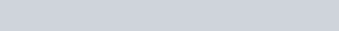Here are the formulas for converting pressure to head: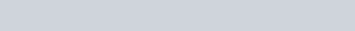• sg. = specific gravity

The last formula you need to know is how velocity converts to head because the only thing a pump can do is impart velocity to the fluid. Since most pumps run at one speed, the pump is a constant velocity device.

Here is how to convert velocity to head: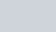• g= the velocity of gravity. 32.2 feet/ second2 or 9,8 meters per second2

You do not need to know Bernoulli’s equation to understand pumps, but it would be good information to have to help you understand the hydraulics inside the pump and its piping. Bernoulli said that assuming no flow loses, “the total head is the same for any point along a stream”.

The formula looks like this in USCS units: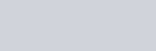In metric units it looks like this: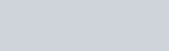The individual terms have the following meanings:

• 144/y = static pressure head (0.102p/in metric)
• C2/2g = dynamic head. “C” is the velocity in this equation
• y = elevation as measured from the pump inlet centerline.

### Posted

• On February 17, 2018